## Example Questions

2 Next →

### Example Question #115 : Trigonometry

In a given right triangle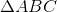, hypotenuse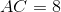and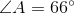. Using the definition of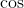, find the length of leg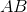. Round all calculations to the nearest hundredth.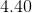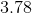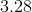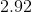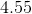Explanation:

In right triangles, SOHCAHTOA tells us that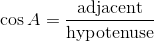, and we know that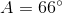and hypotenuse. Therefore, a simple substitution and some algebra gives us our answer.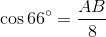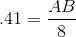Use a calculator or reference to approximate cosine.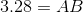Isolate the variable term.

Thus,.

2 Next →